Next: Model of Hipparchus Up: Geometric Planetary Orbit Models Previous: Introduction

# Model of Kepler

Kepler's geometric model of a heliocentric planetary orbit is summed up in his three well-known laws of planetary motion. According to Kepler's first law, all planetary orbits are ellipses which are confocal with the sun and lie in a fixed plane. Moreover, according to Kepler's second law, the radius vector which connects the sun to a given planet sweeps out equal areas in equal time intervals.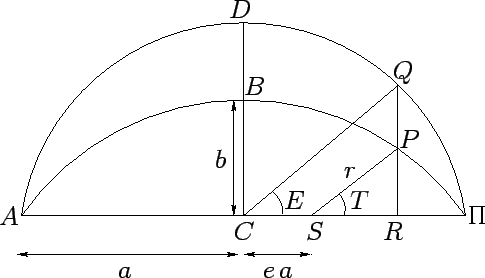Consider Figure 17.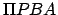is half of an elliptical planetary orbit. Furthermore,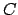is the geometric center of the orbit,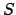the focus at which the sun is located,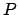the instantaneous position of the planet,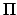the perihelion point (i.e., the planet's point of closest approach to the sun), and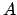the aphelion point (i.e., the point of furthest distance from the sun). The ellipse is symmetric about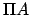, which is termed the major axis, and about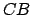, which is termed the minor axis. The length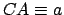is called the orbital major radius. The length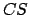represents the displacement of the sun from the geometric center of the orbit, and is generally written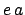, where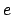is termed the orbital eccentricity, where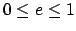. The lengthis called the orbital minor radius. The length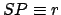represents the radial distance of the planet from the sun. Finally, the angle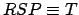is the angular bearing of the planet from the sun, relative to the major axis of the orbit, and is termed the true anomaly.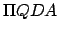is half of a circle whose geometric center is, and whose radius is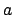. Hence, the circle passes through the perihelion and aphelion points.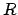is the point at which the perpendicular frommeets the major axis. The point where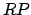produced meets circleis denoted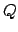. Finally, the angle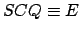is called the elliptic anomaly.

Now, the equation of the ellipseis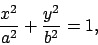(60)

where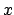and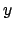are the perpendicular distances from the minor and major axes, respectively. Likewise, the equation of the circleis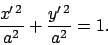(61)

Hence, if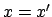then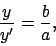(62)

and it follows that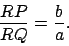(63)

Now,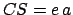. Furthermore, it is easily demonstrated that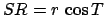,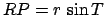,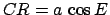, and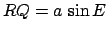. Consequently, Eq. (63) yields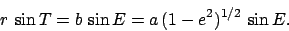(64)

Also, since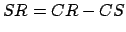, we have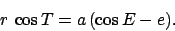(65)

Taking the square root of the sum of the squares of the previous two equations, we obtain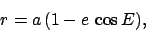(66)

which can be combined with Eq. (65) to give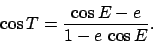(67)

Now, according to Kepler's second law,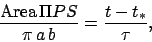(68)

whereis the time at which the planet passes point,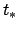the time at which it passes the perihelion point, and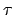the orbital period. However,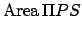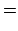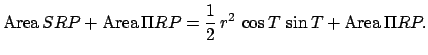(69)

But,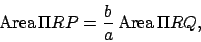(70)

since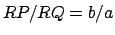for all values of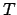. In addition,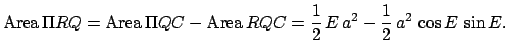(71)

Hence, we can write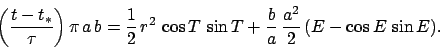(72)

According to Eqs. (64) and (65),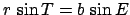, and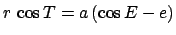, so the above expression reduces to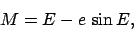(73)

where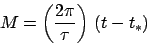(74)

is an angle which is zero at the perihelion point, increases uniformly in time, and has a repetition period which matches the period of the planetary orbit. This angle is termed the mean anomaly.

In summary, the radial and angular polar coordinates,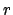and, respectively, of a planet in a Keplerian orbit about the sun are specified as implicit functions of the mean anomaly, which is a linear function of time, by the following three equations: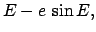(75)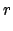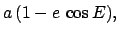(76)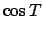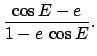(77)

It turns out that the earth and the five visible planets all possess low eccentricity orbits characterized by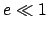. Hence, it is a good approximation to expand the above three equations usingas a small parameter. To second-order, we get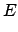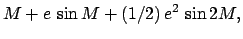(78)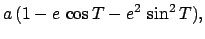(79)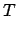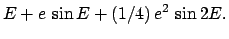(80)

Finally, these equations can be combined to giveandas explicit functions of the mean anomaly: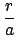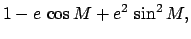(81)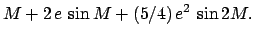(82)Next: Model of Hipparchus Up: Geometric Planetary Orbit Models Previous: Introduction
Richard Fitzpatrick 2010-07-21